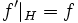# Function extension expression

This page describes a formal expression, or formalism, that can be used to describe certain subgroup properties.

View a complete list of formal expressions for subgroup properties OR View subgroup properties expressible using this formalism

## Definition

### Main definition

A function extension expression is the expression of a subgroup property in terms of two properties of functions (by function here is meant a function from a group to itself). The function extension expression corresponding to function properties$a$ and$b$ is denoted as:$a \leftarrow b$

meaning that$H$ satisfies the property in$G$ if every function$f$ satisfying$b$ on$H$, there exists a function$f'$ satisfying$a$ in$G$, and such that$f'|_H = f$.

The property on the left of the arrow is termed the left side of the function extension expression, and the property on the right side of the arrow is termed the right side of the function extension expression.

## Expressing subgroup properties this way

### Subgroup properties that can be expressed

A subgroup property that can be expressed using a function extension expression is termed a function-extension-expressible subgroup property. A list of all the subgroup properties that are function-extension-expressible is available at Category:Function-extension-expressible subgroup properties.